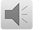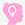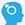# 手把手教你用R语言评价临床预测模型，一文就够（附代码）

•时间：2020-08-03   阅: 62177
标签: 统计方法   数学模型（二）临床模型评价

当临床预测模型成功建立，就会面临一个问题，如何去评价模型的质量。模型的评价一般是通过比较人群的模型预测结果与实际观测结果，来评价预测模型的效果。

对于模型的评价指标，我们往往分成三种：

1. 区分度评价指标：C指数(C-Index)，重新分类指数(Net reclassification index，NRI);

2. 一致性评价指标：校正曲线(Calibration plot);

3. 临床有效性评价指标：决策分析曲线(Decision Curve Analysis， DCA);

今天就让阿琛来给大家分别讲讲如何评价前期建立的临床预测模型。

1.建立预测模型

首先，我们一起快速的回顾一下如何构建Cox回归模型。

1.1 引用R包

#install.packages("rms")

#install.packages("foreign")

#install.packages("survival")

library(rms)

library(foreign)

library(survival)

1.2 读取文件

setwd("C:Users00Desktop13_clinical") #设置工作目录在该数据集中，共包含6个不同的临床病理特征以及患者的生存状态和生存时间。

1.3 构建Cox回归模型

首先，对数据的相关参数进行整理与设置：

rt\$gender <- factor(rt\$gender,labels=c("F", "M"))

rt\$stage <- factor(rt\$stage,labels=c("Stage1", "Stage2", "Stage3", "Stage4"))

rt\$T <- factor(rt\$T,labels=c("T1", "T2", "T3", "T4"))

rt\$M <- factor(rt\$M,labels=c("M0", "M1"))

rt\$N <- factor(rt\$N,labels=c("N0", "N1", "N2", "N3"))

rt\$risk <- factor(rt\$risk,labels=c("low", "high"))

str(rt)

units(rt\$futime) <- "year" #定义时间的单位接着，将所有变量纳入模型中，构建Cox回归模型：

#构建回归模型

f <- cph(Surv(futime, fustat) ~gender + stage +T + M + N + risk,

x=T, y=T, surv=T, data=rt)

f #查看模型的相关参数自此，我们的Cox回归模型就构建完成了。接下来就是从不同的角度来评价模型的质量。

2.C指数(C-index)的计算

validate(f, method="boot", B=1000, dxy=T) #重复模拟1000次

rcorrcens(Surv(futime, fustat) ~ predict(f), data = rt)

结果显示：在此模型中，C-index为0.664，即1-0.336=0.664。

当然，根据C指数而言，该模型的预测区分度并不是太高。一般而言，我们将0.51-0.7认为是低可信度，0.71-0.9为中等可信度，> 0.9为高可信度。

3.Calibration plot的绘制

绘制三年生存率的校准曲线

#计算三年生存率的校准曲线

f3 <- cph(Surv(futime, fustat) ~gender + stage +T + M + N + risk,

x=T, y=T, surv=T, time.inc = 3, data=rt)

cal3 <- calibrate(f3, cmethod="KM", method="boot", u= 3, m= 90, B= 1000)

#一般将患者分成3组，即m约等于患者总数/3，且重复模拟1000次

plot(cal3)

结果显示：绘制五年生存率的校准曲线

#计算五年生存率的校准曲线

f5 <- cph(Surv(futime, fustat) ~gender + stage +T + M + N + risk,

x=T, y=T, surv=T, time.inc = 5, data=rt)

cal5 <- calibrate(f5, cmethod="KM", method="boot", u= 5, m= 90, B= 1000)

plot(cal5)

结果显示：对于Calibration plot，横坐标为模型预测得到的患者生存率，纵坐标为患者实际观察得到的生存率，三点之间的联系与虚线之间越相近，越能说明模型良好的一致性。

4.DCA曲线的绘制：

source("stdca.R") #把stdca.R放在之前设定的工作目录中，并进行引用

rt\$three.years.Survival.Probabilitynew = c(1- (summary(survfit(f, newdata = rt),times = 3)\$surv)) #计算3年生存率

rt\$five.years.Survival.Probabilitynew = c(1- (summary(survfit(f, newdata = rt),times = 5)\$surv)) #计算5年生存率

write.csv(rt, "DCA.Survival.csv")

#将患者的3年和5年生存率结果保存

随后，根据患者的生存情况，分别绘制3年和5年的DCA曲线;

#绘制3年生存率的DCA曲线

stdca(data=rt,

outcome="fustat", ttoutcome="futime", timepoint=3,

predictors="three.years.Survival.Probabilitynew",

xstop=0.9, smooth=TRUE)

结果显示：#绘制5年生存率的DCA曲线

stdca(data=rt,

outcome="fustat", ttoutcome="futime", timepoint=5,

predictors="five.years.Survival.Probabilitynew",

xstop=0.9,smooth=TRUE)

结果显示：5.NRI指数的计算

对于NRI指数的计算，有2个专门的R包，分别为nricens和PredictABEL;其中，nricens计算出来的是绝对的NRI，PredictABEL则为相对的NRI，一般在使用过程中选择其中之一即可。

#install.packages("nricens")

library(nricens)

随后，分别构建两个不同的模型，以进行比较：

mstd <- cph(Surv(futime, fustat) ~gender + stage +T + M + N,

x=T, y=T, surv=T, data=rt) #使用除risk外的变量进行建模

mnew <- cph(Surv(futime, fustat) ~gender + stage +T + M + N + risk,

x=T, y=T, surv=T, data=rt) #该模型包含所有的变量

接着，计算NRI指数;

#计算连续的NRI，取值为5%时就定义为重分类

#3年生存率NRI

nricens(mdl.std = mstd, mdl.new = mnew, t0 = 3, updown = 'diff',cut = 0.05, niter = 200)

#5年生存率NRI

nricens(mdl.std = mstd, mdl.new = mnew, t0 = 5, updown = 'diff',cut = 0.05, niter = 200)

#计算分类变量计算的NRI

nricens(mdl.std = mstd, mdl.new = mnew, t0 = 5, updown = 'category',cut = c(0.3,0.6), niter = 200)

对于NRI的计算，其主要是比较新旧两个模型之间存在区分度。其中，updown主要定义了一个样本的风险是否变动的方式，category是指分类值，即熟悉的低、中、高风险，另有一种diff，为连续值。

Cut是判断风险高低的临界值。当updown为diff时，cut只需设置1个值，比如0.05，即认为当预测的风险在新旧模型中相差5%时，即被认为是重新分类了;而当updown为category时，可以对cut设置两个不同的值，即0~29%为低风险，30%~59%为中风险，60%~100%为高风险。

结果显示：当updown为diff时，3年和5年的NRI值分别为0.26和0.53，均大于5%，可以认为预测的风险在新旧模型中发生了重新分类;

3年生存率NRI的95%可信区间：5年生存率NRI的95%可信区间：同时，由于做了200次重复取样，相当于有200个NRI，于是NRI就有了标准误和95%的置信区间。同理，可以得到当updown为category时的NRI值。

到此，对于临床预测模型评价指标的介绍就到此结束了。大家可以对照着整个分析过程，使用示例数据或者自己的模型数据，进行相应的学习~